# Test: Diode Circuits- 2

## 20 Questions MCQ Test Topicwise Question Bank for Electrical Engineering | Test: Diode Circuits- 2

Description
Attempt Test: Diode Circuits- 2 | 20 questions in 60 minutes | Mock test for Electrical Engineering (EE) preparation | Free important questions MCQ to study Topicwise Question Bank for Electrical Engineering for Electrical Engineering (EE) Exam | Download free PDF with solutions
QUESTION: 1

### For the network shown below, the input swing of 30 volts is equal to the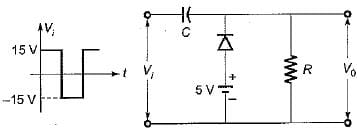Solution:

• During the negative half cycle of input voltage Vi, diode is forward biased and acts as short-circuit. Hence capacitor changes to VC volts.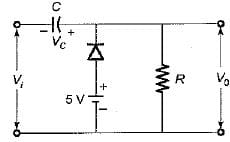Using KVL,
-5 + VC - 15 = 0
or,  VC - 20 volts
Hence, V0 = 5 volts
• During the positive half cycle of input voltage Vi, diode is reverse biased and acts as open-circuit.
Applying KVL, we have
15 = -20 + \/0
or V0 = 35 volts
The waveforms for Vi and V0 will be as shown below: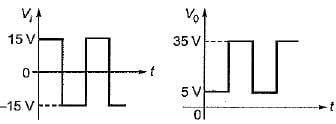Thus, the input swing of 30 V is equal to the output swing of 30 V.

QUESTION: 2

### The output voltage V0 for the circuit shown below for the given sinusoidal input signal will be represented as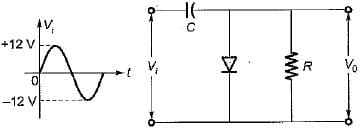Solution:

• When Vi is positive:
Diode is ON and acts as short circuit. Output voltage,
V0 = 0V
The capacitor charges to 12 volts as shown below and acts like a battery.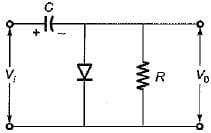• When Vi is negative:
Diode is OFF and acts as open circuit. Output voltage,
V0 = -12-12 = -24 Volt
This gives negatively clamped voltage and the resultant output waveform will be as shown in figure below.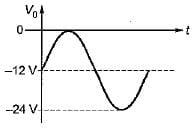QUESTION: 3

### Match List-I (Circuits) with List-II (Applications) and select the correct answer using the codes given below the lists: List-I A. Diode comparators B. Diode dampers C. Astable multivibrator D. Diode clippers List-II 1. Slicer 2. Oscillator 3. DC level to an AC signal 4. Square wave from a sine wave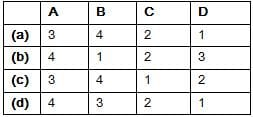Solution:
QUESTION: 4

The circuit shown below represents a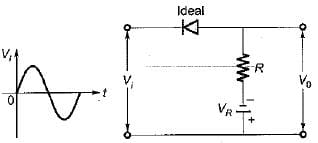Solution:

• WhenVi is positive:
Diode is OFF so that, V0 = -VR
• When Vi is negative: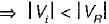Diode is OFF so that,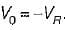.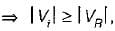Diode is ON so that,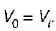Hence, output waveform will be as shown below.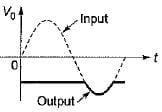QUESTION: 5

Assertion (A): Clipping circuit controls the shape of the waveform by clipping or removing a portion of the applied wave.
Reason (R): It needs minimum three components namely an ordinary diode, a resistor and a capacitor.

Solution:

A clamper circuit requires a capacitor not a clipping circuit. Hence, reason is not a correct statement.

QUESTION: 6

Consider the following statements associated with the diode rectifier circuits:
1. The maximum reverse voltage, which can be applied before the breakdown point is reached, is called the “peak inverse voltage”.
2. In a full-wave rectifier, the current in each of the diode flows for the whole input signal cycle.
3. The ratio of direct or average value of the output to the effective value of AC component present in the rectifier output is called the “ripple factor”.
4. Availability of low-cost silicon diodes has made bridge circuit arrangement more economical than centre trapped transformer arrangement despite its requirement of four diodes:

Q. Which of the above statements are correct?

Solution:

• In a full-wave rectifier each diode conducts during one half-cycle. Hence, statement-2 is not correct.
• Ripple factor is the ratio of rms value of AC component to the DC component in the output.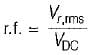Hence, statement - 3 is also not correct.

QUESTION: 7

In the Zener regulator shown below, the output requirements are 5 V, 20 mA. Iz(min) and Iz(max) are 5 mA and 80 mA, then the value of limiting resistor (R) and the load resistance (RL) will be respectively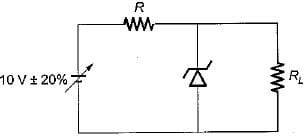Solution:

Given, minimum Zener current = Iz(min) = 5 mA when the input voltage is minimum.
Here, the input voltage varies between 8 V to 12V.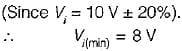Given, lL = 20 mA and voltage across load,
V0 = 5 volts
∴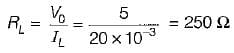∴ Limiting resistance,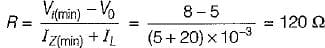QUESTION: 8

For the zener voltage regular circuit shown below, the output voltage is required to be maintained at 5 volt with a load current of 10 mA. The zener wattage is 400 mW and input voltage is specified as 10 V ± 2 V.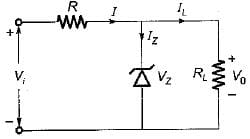One of the possible value of series resistance R will be

Solution:

Given, V0 = 5 V, IL = 10 mA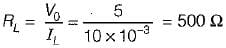Maximum Zener current,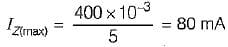(Since Pz(max) = 400 mW)
The minimum input voltage required will be when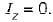Under this condition,
I = IL = 10 mA
Minimum input voltage,
Vi(min) = V0 +IR    ...(i)
Given, Vi = 10 V ± 2 V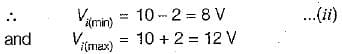From equations (i) and (ii), we have
8 = 5 + (10 x 10-3) R
or,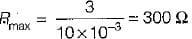Now, maximum input voltage,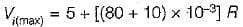or 12 = 5 + (90 x 10-3) R
or,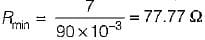Hence, 77.77 Ω < R < 300 Ω
Thus, one of the possible value of out of the given options is R = 266 Ω

QUESTION: 9

The Zener diode VZ1 in the figure shown below has the reverse saturation current of 20 mA and reverse breakdown voltage of 100 V whereas the corresponding values for diode  VZ2  are 40 μA and 40 V.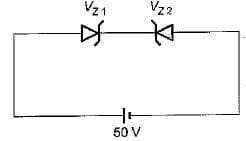The current through the circuit is

Solution:

The Zener diode VZ2 is reverse biased and VZ1 is forward biased. As both Zener diodes \/Z1 and VZ2 are connected in series, the reverse saturation current 40 μA of VZ2 will flow clockwise in the circuit as 50 V reverse bias appears across the VZ2 diode.

QUESTION: 10

Match List-I (Diode Circuits) with List-ll (Output-Input Characteristics) and select the correct answer using the codes given below the lists:
List-I
A.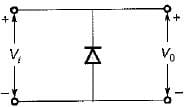B.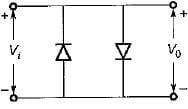C.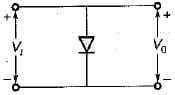List-II
1.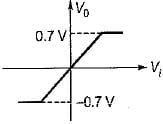2.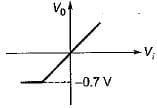3.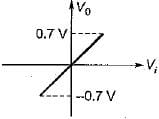4.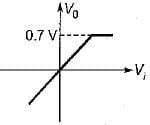Codes: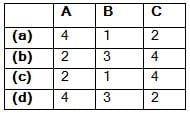Solution:
QUESTION: 11

What is the output voltage of clipper circuit shown in figure below for the sinusoidal input voltage with its peak voltage of 100 V, assuming both diodes ideal?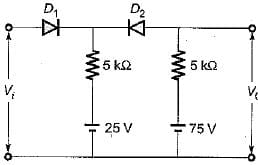Solution:

For, V< 25 volts,
Diode D1 will be OFF while diode D2 will be ON.
∴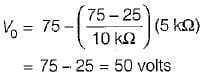QUESTION: 12

For the circuit shown below, assume that the diodes are ideal. The meter reading would be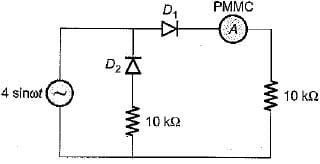Solution:

The PMMC ammeter will read average value of current. The current through the PMMC ammeter will flow only for positive half cycle of input voltage while current through it will be zero for the negative half cycle.
Hence,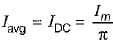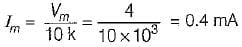∴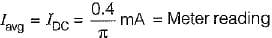QUESTION: 13

Two identical diodes, D1 and D2 are connected back to back as shown in figure below. The reverse saturation current I0 of each diode is 10-8 A and the breakdown voltage is 50 V. The voltage drop across diodes D1 and D2 will be respectively (assume kTlq = 25 mV)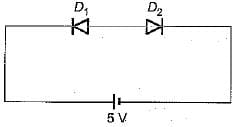Solution:

Diode D1 is reverse biased and diode D2 is forward biased, but as both the diodes form a close loop, the reverse saturation current flows through both the diodes.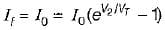or,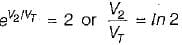or, V2 = VTln 2 = (25 mV) x In 2
= 25 x10-3 x 0 .693
= 17.33 mV = 0.01733 V
∴ Voltage drop across D2 is
v2 ≈ 0.0173 volt
and voltage drop across D1 is
V1 ≈ 5 - 0.0173 ≈ 4.983 volts

QUESTION: 14

The 6 V Zener diode shown in figure has zero zener resistance and a knee current of 5 mA.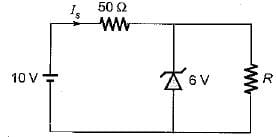The minimum value of R so that the voltage across it does not fall below 6 V is

Solution: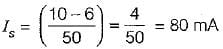The maximum load current = 80 - 5 = 75 mA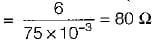QUESTION: 15

What are the values of V0 and I for the diode circuit shown below?
(Assume the drop across the diodes to be 0.7 V)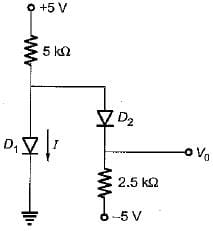Solution:

If the diode D1 conducts, the current I must flow as indicated in figure below.
Here,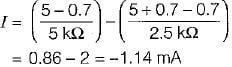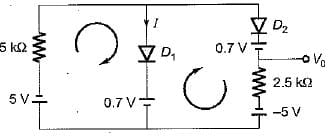Since, I is negative, therefore D1 does not conduct.
∴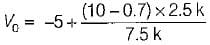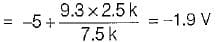QUESTION: 16

A DC power supply has no-load voltage of 30 V, and a full-load voltage of 25 V at a full-load current of 1 A. Its output resistance and load regulation, respectively, are

Solution: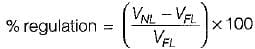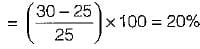Output resistance =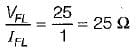QUESTION: 17

Higher order active filters are used for variable

Solution:

Higher order active filters are used for variable roll-off rate.

QUESTION: 18

In the figure shown below, the diode of Op-amps are ideal. For an input Vin = sin (ωt) the output voltages V0 is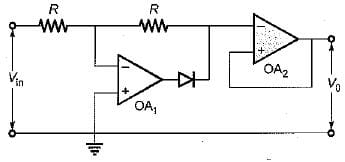Solution:
QUESTION: 19

The cutin voltage for each diode in figure is Vγ = 0.6 V. Each diode current is 0.5 mA. The value of R1 R2 and R3 will be respectively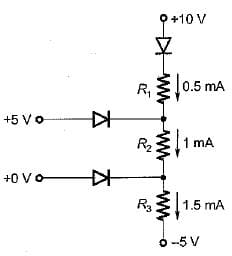Solution: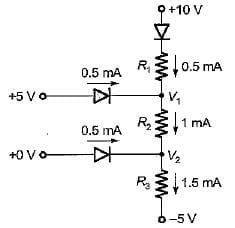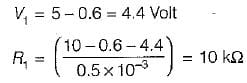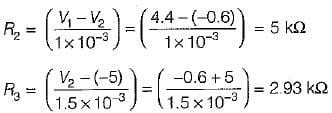(∵ V2 = 4.4 - 5 = - 0.6 volt)

QUESTION: 20

In the circuit shown below, diodes D1 and D2 are ideal. The current i1 and i2 are respectively​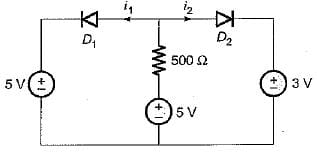Solution: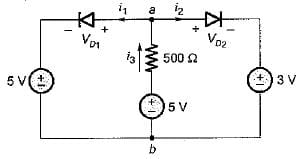For i3 > 0, Vab < 5 volts and VD1 < 0
Hence, i = 0
and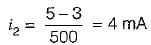Use Code STAYHOME200 and get INR 200 additional OFF Use Coupon Code